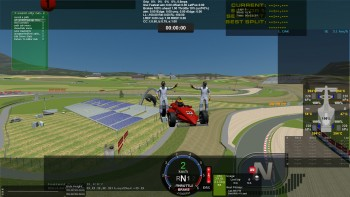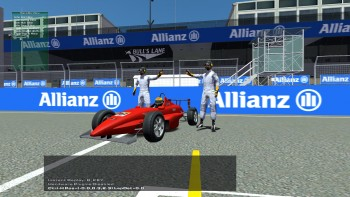# [SOLVED] How to fix car point in air?

Discussion in 'Track Modding' started by DA, Oct 27, 2014.

1. ### DARegistered

Joined:
Oct 5, 2010
Messages:
261
Likes Received:
6
Hey guys, I convert Red Bull Ring from new game, but when i loading on the track, i spawned in AIR. How to fix it?Cheers,
DA.

Step by step for fix this problem!

1. Set new 0,0,0 in 3DSimED!

How do it?

1. Import your scn file.
2. Press Ctrl+A→Check objects→Move→Set new coords for 0,0,0 point!
3. Set new coords in your start/finish line!

Then you need SET material for your road! How do it? All terains in tdf file!

1. Highlight the texture of the road. Example my texture was named act_tarmac_a.
2. Tools → Rename Materials
3. Find there 'your road texture name' and change it for 'roada'.
4. Export your new scn and check it in game!

Please read note!

road - is default rFactor 2 terrain name. You can use roadtexture, roada, roadb, roadwet etc!

Results after fix:Special regards for Kristoff Rand and Hazi for help!

Last edited by a moderator: Oct 28, 2014
2. ### Kristoff RandRegistered

Joined:
Dec 23, 2011
Messages:
1,055
Likes Received:
10
You're not spawning in the air... your units were wrong when you converted. the car is far, far, far too large.

3. ### DARegistered

Joined:
Oct 5, 2010
Messages:
261
Likes Received:
6
And what i can do for fix it?Maybe some manuals or simple todo list?4. ### Kristoff RandRegistered

Joined:
Dec 23, 2011
Messages:
1,055
Likes Received:
10
My best help would be to say to check the scale units that the model was built in and make sure to use them or convert them properly...

or find the the exact size of a part of the car, like the wheel base and then scale your model to that measurement in whatever program you're using to model.

I would need to know what software you're using to make your model and your track? what units are they set to?

I would need to know more about the model and what units it was made for? meter? millimeter? inches? and what unit you imported it into?

5. ### DARegistered

Joined:
Oct 5, 2010
Messages:
261
Likes Received:
6
3DSimED 3.0u.Car standart from SDK rF2 860.

6. ### HaziRegistered

Joined:
Jan 15, 2012
Messages:
801
Likes Received:
89
Maybe just mark whole track in 3DSimed and move it so that the Start/Finish (lowest part of circuit) is at the 0/0/0 point coordinates of 3DSimed and export again. You just swap the gmt files in the mas file and all other files stay the same.

7. ### DARegistered

Joined:
Oct 5, 2010
Messages:
261
Likes Received:
6
Hazi, i check it!But first view - it works! Next report later.How to do?

3DSimED 0.3u

1. Import _output.scn
2. Press Ctrl+A→Check objects→Move→Set new coords for 0,0,0 point!
3. Soon.8. ### DARegistered

Joined:
Oct 5, 2010
Messages:
261
Likes Received:
6
9. ### HaziRegistered

Joined:
Jan 15, 2012
Messages:
801
Likes Received:
89
Might be a Problem with the tdf file - check if the materials for the drivin surface matches the entries in the tdf file - and (re)name the materials like in the tdf from a newer original RF2 track - Sao Paulo - you find it under Tools - rename materials - in 3DSimed

[TRACKVARS]
RoadDryGrip=1.00
RoadWetGrip=0.85
RoadmetalGrip=0.80
RoadDustGrip=0.90
RoadBumpAmp=0.001
RoadBumpLen=3.0
RumbleDryGrip=0.96
RumbleWetGrip=0.60
RumbleBumpAmp=0.001
RumbleBumpLen=3.0
MiscBumpAmp=0.07
MiscBumpLen=4.0
HATFilterMaxOffset=0.0015

////////////////////////////////////////////////////ROADS////////////////////////////////////////////////////////

// Roads
[FEEDBACK]
Wear=1.05 Dry=1.02 Wet=0.85 Roughness=(0.50,0.30) Resistance=0.0 BumpAmp=RoadBumpAmp BumpWavelen=RoadBumpLen Legal=true Spring=0.0 Damper=0.0 CollFrict=0.4 Sparks=1 Scraping=1 Sound=dry
Reaction=tiresmoke Tex=SMOKETire.tga Max=1024 Scale=(0.5,0.5,0.5) Growth=(2.5,2.0,2.0) ASDEnvelope=(0.1,0.7,3.8) Suspension=0.98 DestBlend=InvSrcAlpha SrcBlend=SrcAlpha
Reaction=skid Tex=skidhard.tga Max=2500 Pixel=NoReduceDetail Particle=Plane+Deformable+SingleSided DestBlend=InvSrcAlpha SrcBlend=SrcAlpha
Reaction=wetskid Tex=skidwet.tga Max=1024 Duration=0.40 Pixel=NoReduceDetail Particle=Plane+Deformable+SingleSided DestBlend=One SrcBlend=SrcAlpha
Reaction=spray Tex=rainspray.tga Max=1024 Scale=(1.3,0.02,0.02) Growth=(2.0,0.19,0.19) GrowthVel=(0.17,0.13,0.13) Power=0.41 RampSpeed=90.0 OffsetVel=0.10 ASDEnvelope=(0.01,3.0,2.0) DestBlend=InvSrcAlpha SrcBlend=SrcAlpha
Materials=road

// Roads
[FEEDBACK]
Wear=1.00 Dry=0.99 Wet=0.79 Roughness=(0.50,0.25) Resistance=0.0 BumpAmp=0.001 BumpWavelen=RoadBumpLen Legal=true Spring=0.0 Damper=0.0 CollFrict=0.4 Sparks=1 Scraping=1 Sink=-0.0015 Sound=dry
Reaction=tiresmoke Tex=SMOKETire.tga Max=1024 Scale=(0.5,0.5,0.5) Growth=(2.5,2.0,2.0) ASDEnvelope=(0.1,0.7,3.8) Suspension=0.98 DestBlend=InvSrcAlpha SrcBlend=SrcAlpha
Reaction=skid Tex=skidhard.tga Max=2500 Pixel=NoReduceDetail Particle=Plane+Deformable+SingleSided DestBlend=InvSrcAlpha SrcBlend=SrcAlpha
Reaction=wetskid Tex=skidwet.tga Max=1024 Duration=0.40 Pixel=NoReduceDetail Particle=Plane+Deformable+SingleSided DestBlend=One SrcBlend=SrcAlpha
Reaction=spray Tex=rainspray.tga Max=1024 Scale=(1.3,0.02,0.02) Growth=(2.0,0.19,0.19) GrowthVel=(0.17,0.13,0.13) Power=0.41 RampSpeed=90.0 OffsetVel=0.10 ASDEnvelope=(0.01,3.0,2.0) DestBlend=InvSrcAlpha SrcBlend=SrcAlpha
Materials=rdil

// Abbrasive Roads
[FEEDBACK]
Wear=1.15 Dry=1.04 Wet=0.89 Roughness=(0.50,0.25) Resistance=0.0 BumpAmp=0.001 BumpWavelen=RoadBumpLen Legal=true Spring=0.0 Damper=0.0 CollFrict=0.4 Sparks=1 Scraping=1 Sink=-0.002 Sound=dry
Reaction=tiresmoke Tex=SMOKETire.tga Max=1024 Scale=(0.5,0.5,0.5) Growth=(2.5,2.0,2.0) ASDEnvelope=(0.1,0.7,3.8) Suspension=0.98 DestBlend=InvSrcAlpha SrcBlend=SrcAlpha
Reaction=skid Tex=skidhard.tga Max=2500 Pixel=NoReduceDetail Particle=Plane+Deformable+SingleSided DestBlend=InvSrcAlpha SrcBlend=SrcAlpha
Reaction=wetskid Tex=skidwet.tga Max=1024 Duration=0.40 Pixel=NoReduceDetail Particle=Plane+Deformable+SingleSided DestBlend=One SrcBlend=SrcAlpha
Reaction=spray Tex=rainspray.tga Max=1024 Scale=(1.3,0.02,0.02) Growth=(2.0,0.19,0.19) GrowthVel=(0.17,0.13,0.13) Power=0.41 RampSpeed=90.0 OffsetVel=0.10 ASDEnvelope=(0.01,3.0,2.0) DestBlend=InvSrcAlpha SrcBlend=SrcAlpha
Materials=rdab

// Road Ruts
[FEEDBACK]
Wear=1.02 Dry=1.0 Wet=0.8 Roughness=(0.50,0.25) Resistance=0.0 BumpAmp=0.001 BumpWavelen=RoadBumpLen Legal=true Spring=0.0 Damper=0.0 CollFrict=0.4 Sparks=1 Scraping=1 Sink=0.002 Sound=dry
Reaction=tiresmoke Tex=SMOKETire.tga Max=1024 Scale=(0.5,0.5,0.5) Growth=(2.5,2.0,2.0) ASDEnvelope=(0.1,0.7,3.8) Suspension=0.98 DestBlend=InvSrcAlpha SrcBlend=SrcAlpha
Reaction=skid Tex=skidhard.tga Max=2500 Pixel=NoReduceDetail Particle=Plane+Deformable+SingleSided DestBlend=InvSrcAlpha SrcBlend=SrcAlpha
Reaction=wetskid Tex=skidwet.tga Max=1024 Duration=0.40 Pixel=NoReduceDetail Particle=Plane+Deformable+SingleSided DestBlend=One SrcBlend=SrcAlpha
Reaction=spray Tex=rainspray.tga Max=1024 Scale=(1.3,0.02,0.02) Growth=(2.0,0.19,0.19) GrowthVel=(0.17,0.13,0.13) Power=0.41 RampSpeed=90.0 OffsetVel=0.10 ASDEnvelope=(0.01,3.0,2.0) DestBlend=InvSrcAlpha SrcBlend=SrcAlpha
Materials=rdrut

// Roads with Marbles
[FEEDBACK]
Wear=1.00 Dry=0.98 Wet=0.81 Roughness=(0.50,0.3) Resistance=0.0 BumpAmp=0.006 BumpWavelen=0.3 Legal=true Spring=0.0 Damper=0.0 CollFrict=0.4 Sparks=1 Scraping=1 Sound=dry
Reaction=tiresmoke Tex=SMOKETire.tga Max=1024 Scale=(0.5,0.5,0.5) Growth=(2.5,2.0,2.0) ASDEnvelope=(0.1,0.7,3.8) Suspension=0.98 DestBlend=InvSrcAlpha SrcBlend=SrcAlpha
Reaction=skid Tex=skidhard.tga Max=2500 Pixel=NoReduceDetail Particle=Plane+Deformable+SingleSided DestBlend=InvSrcAlpha SrcBlend=SrcAlpha
Reaction=wetskid Tex=skidwet.tga Max=1024 Duration=0.40 Pixel=NoReduceDetail Particle=Plane+Deformable+SingleSided DestBlend=One SrcBlend=SrcAlpha
Reaction=spray Tex=rainspray.tga Max=1024 Scale=(1.3,0.02,0.02) Growth=(2.0,0.19,0.19) GrowthVel=(0.17,0.13,0.13) Power=0.41 RampSpeed=90.0 OffsetVel=0.10 ASDEnvelope=(0.01,3.0,2.0) DestBlend=InvSrcAlpha SrcBlend=SrcAlpha
Materials=rdmarbles

// Concrete
[FEEDBACK]
Wear=1.1 Dry=0.99 Wet=0.81 Roughness=(0.50,0.25) Resistance=3.0 BumpAmp=0.004 BumpWavelen=1.1 Legal=true Spring=0.0 Damper=0.0 CollFrict=0.4 Sparks=1 Scraping=1 Sink=-0.002 Sound=dry
Reaction=tiresmoke Tex=SMOKETire.tga Max=1024 Scale=(0.5,0.5,0.5) Growth=(2.5,2.0,2.0) ASDEnvelope=(0.1,0.7,3.8) Suspension=0.98 DestBlend=InvSrcAlpha SrcBlend=SrcAlpha
Reaction=skid Tex=skidhard.tga Max=2500 Pixel=NoReduceDetail Particle=Plane+Deformable+SingleSided DestBlend=InvSrcAlpha SrcBlend=SrcAlpha
Reaction=wetskid Tex=skidwet.tga Max=1024 Duration=0.40 Pixel=NoReduceDetail Particle=Plane+Deformable+SingleSided DestBlend=One SrcBlend=SrcAlpha
Reaction=spray Tex=rainspray.tga Max=1024 Scale=(1.3,0.02,0.02) Growth=(2.0,0.19,0.19) GrowthVel=(0.17,0.13,0.13) Power=0.41 RampSpeed=90.0 OffsetVel=0.10 ASDEnvelope=(0.01,3.0,2.0) DestBlend=InvSrcAlpha SrcBlend=SrcAlpha
Materials=conc

// Paint
[FEEDBACK]
Wear=0.85 Dry=0.88 Wet=0.50 Roughness=(0.45,0.02) Resistance=0 BumpAmp=0.002 BumpWavelen=5 Legal=true Spring=0 Damper=0 CollFrict=0.2 Sparks=1 Scraping=1 OnTop=0.0015 Sound=dry
Reaction=tiresmoke Tex=SMOKETire.tga Max=1024 Scale=(0.5,0.5,0.5) Growth=(2.5,2.0,2.0) ASDEnvelope=(0.1,0.7,3.8) Suspension=0.98 DestBlend=InvSrcAlpha SrcBlend=SrcAlpha
Reaction=skid Tex=skidhard.tga Max=2500 Pixel=NoReduceDetail Particle=Plane+Deformable+SingleSided DestBlend=InvSrcAlpha SrcBlend=SrcAlpha
Reaction=wetskid Tex=skidwet.tga Max=1024 Duration=0.40 Pixel=NoReduceDetail Particle=Plane+Deformable+SingleSided DestBlend=InvSrcAlpha SrcBlend=SrcAlpha
Reaction=spray Tex=rainspray.tga Max=1024 Scale=(1.3,0.02,0.02) Growth=(2.0,0.19,0.19) GrowthVel=(0.17,0.13,0.13) Power=0.41 RampSpeed=70 OffsetVel=0.10 ASDEnvelope=(0.01,3.0,2.0) DestBlend=InvSrcAlpha SrcBlend=SrcAlpha
Materials=strp

///////////////////////////////////////////////Dirt and Grass////////////////////////////////////////////////

// Grass
[FEEDBACK]
Wear=0.6 Dry=0.67 Wet=0.35 Resistance=2500 BumpAmp=0.035 BumpWavelen=1.5 Legal=false Spring=300.0 Damper=300.0 CollFrict=2.2 Sparks=0 Scraping=0 Sink=0.035 Sound=grass
Reaction=softskid Tex=skidgreen.tga Max=1024 Pixel=NoReduceDetail Particle=Plane+Deformable+SingleSided DestBlend=InvSrcAlpha SrcBlend=SrcAlpha
Reaction=dust Tex=DIRT_Cloud.tga Max=128 TopSpeed=105.0 Scale=(1.4,1.0,1.0) Growth=(3.0,2.0,2.0) Suspension=0.847 ASDEnvelope=(1.0,1.2,3.5) DestBlend=InvSrcAlpha SrcBlend=SrcAlpha
Reaction=dirt Tex=GrassDirtKick.tga Max=64 Scale=(0.7,0.7,0.7) Growth=(0.85,0.85,0.85) Suspension=0.693 ASDEnvelope=(0.2,0.8,0.2) DestBlend=InvSrcAlpha SrcBlend=SrcAlpha
Materials=gras

// Gravel
[FEEDBACK]
Wear=0.80 Dry=0.7 Wet=0.5 Resistance=40000 BumpAmp=0.063 BumpWavelen=1.0 Legal=false Spring=400.0 Damper=200.0 CollFrict=5.0 Sparks=0 Scraping=0 Sink=0.05 Sound=gravel
Reaction=dust Tex=DIRT_Cloud.tga Max=512 TopSpeed=80.0 Scale=(1.0,1.0,1.0) Growth=(3.0,2.0,2.0) Suspension=0.987 ASDEnvelope=(0.1,2.0,3.5) DestBlend=InvSrcAlpha SrcBlend=SrcAlpha
Reaction=dirt Tex=GravelKick.tga Max=64 Scale=(0.7,0.7,0.7) Growth=(0.9,0.75,0.75) Suspension=0.7 ASDEnvelope=(0.01,2.1,0.01) DestBlend=InvSrcAlpha SrcBlend=SrcAlpha
Reaction=softskid Tex=skidbrown.tga Max=1024 Pixel=NoReduceDetail Particle=Plane+Deformable+SingleSided DestBlend=InvSrcAlpha SrcBlend=SrcAlpha
Materials=grvl, sand, gravel

//////////////////////////////////////////////////RUMBLE STRIPS//////////////////////////////////////////////////////

// Rumblestrips
[FEEDBACK]
Wear=0.96 Dry=0.93 Wet=0.60 Roughness=(0.46,0.16) Resistance=0 BumpAmp=0.002 BumpWavelen=3 Legal=true Spring=0 Damper=0 CollFrict=0.4 Sparks=0 Scraping=1 Sink=-0.001 Sound=rumble
Reaction=tiresmoke Tex=SMOKETire.tga Max=1024 Scale=(0.5,0.5,0.5) Growth=(2.5,2.0,2.0) ASDEnvelope=(0.1,0.7,3.8) Suspension=0.98 DestBlend=InvSrcAlpha SrcBlend=SrcAlpha
Reaction=skid Tex=skidhard.tga Max=2500 Pixel=NoReduceDetail Particle=Plane+Deformable+SingleSided DestBlend=InvSrcAlpha SrcBlend=SrcAlpha
Reaction=wetskid Tex=skidwet.tga Max=1024 Duration=0.40 Pixel=NoReduceDetail Particle=Plane+Deformable+SingleSided DestBlend=InvSrcAlpha SrcBlend=SrcAlpha
Reaction=spray Tex=rainspray.tga Max=1024 Scale=(1.3,0.02,0.02) Growth=(2.0,0.19,0.19) GrowthVel=(0.17,0.13,0.13) Power=0.41 RampSpeed=70 OffsetVel=0.10 ASDEnvelope=(0.01,3.0,2.0) DestBlend=InvSrcAlpha SrcBlend=SrcAlpha
Materials=rmbga

// Painted Smooth Curbs
[FEEDBACK]
Wear=0.98 Dry=0.86 Wet=0.50 Roughness=(0.46,0.15) Resistance=0 BumpAmp=RoadBumpAmp BumpWavelen=RoadBumpLen Legal=false Spring=0 Damper=0 CollFrict=0.4 Sparks=1 Scraping=1 Sink=-0.001 Sound=dry
Reaction=tiresmoke Tex=SMOKETire.tga Max=1024 Scale=(0.5,0.5,0.5) Growth=(2.5,2.0,2.0) ASDEnvelope=(0.1,0.7,3.8) Suspension=0.98 DestBlend=InvSrcAlpha SrcBlend=SrcAlpha
Reaction=skid Tex=skidhard.tga Max=2500 Pixel=NoReduceDetail Particle=Plane+Deformable+SingleSided DestBlend=InvSrcAlpha SrcBlend=SrcAlpha
Reaction=wetskid Tex=skidwet.tga Max=1024 Duration=0.40 Pixel=NoReduceDetail Particle=Plane+Deformable+SingleSided DestBlend=InvSrcAlpha SrcBlend=SrcAlpha
Reaction=spray Tex=rainspray.tga Max=1024 Scale=(1.3,0.02,0.02) Growth=(2.0,0.19,0.19) GrowthVel=(0.17,0.13,0.13) Power=0.41 RampSpeed=70 OffsetVel=0.10 ASDEnvelope=(0.01,3.0,2.0) DestBlend=InvSrcAlpha SrcBlend=SrcAlpha
Materials=rmbla, rmblc

// Crete (fake bumping added)
[FEEDBACK]
Wear=0.70 Dry=0.7 Wet=0.40 Resistance=100 BumpAmp=0.009 BumpWavelen=1.2 Legal=False Spring=100 Damper=100 CollFrict=0.1 Sparks=1 Scraping=1 Sink=0.008 Sound=grass
Reaction=softskid Tex=SkidTURF.tga Max=1024 Pixel=NoReduceDetail Particle=Plane+Deformable+SingleSided DestBlend=InvSrcAlpha SrcBlend=SrcAlpha
Reaction=dust Tex=TurfKICK.tga Max=512 TopSpeed=150 Scale=(1.0,1.0,1.0) Growth=(3.0,2.0,2.0) Suspension=0.99 ASDEnvelope=(0.1,2.0,1.5) DestBlend=InvSrcAlpha SrcBlend=SrcAlpha
Materials=crete

/////////////////////////////////////////////////////WALLS/////////////////////////////////////////////////////////

// Tirewalls
[FEEDBACK]
Legal=false Spring=5000.0 Damper=500.0 CollFrict=2.00 Sparks=0 Scraping=0 WallSkids=1 InsideColl=Secondary\haybale_in OutsideColl=Secondary\haybale_ex
Materials=twal

// Hay Bayles
[FEEDBACK]
Legal=false Spring=7000.0 Damper=400.0 CollFrict=0.80 Sparks=0 Scraping=0 WallSkids=1 InsideColl=Secondary\haybale_in OutsideColl=Secondary\haybale_ex
Materials=HayB

// Cones
[FEEDBACK]
Legal=false Spring=7000.0 Damper=400.0 CollFrict=0.80 Sparks=0 Scraping=0 WallSkids=1 InsideColl=Secondary\haybale_in OutsideColl=Secondary\haybale_ex
Materials=cone

// Woodwalls
[FEEDBACK]
Legal=false Spring=1000.0 Damper=4500.0 CollFrict=0.90 Sparks=0 Scraping=1 WallSkids=1 InsideColl=Secondary\haybale_in OutsideColl=Secondary\haybale_ex
Materials=wwal

// Cementwalls (spring/damper = 0 for immovable objects)
[FEEDBACK]
Legal=false Spring=0.0 Damper=0.0 CollFrict=0.18 Sparks=0 whitesmoke=1 Scraping=1 Wallskids=1 Sound=solidwall
Materials=cem,wall,cmwl

// Guardrails (spring/damper = 0 for immovable objects)
[FEEDBACK]
Legal=false Spring=0.0 Damper=0.0 CollFrict=0.10 Sparks=1 Scraping=1 Wallskids=1 Sound=guardrail
Materials=grdr

// SAFER Walls (spring/damper = 0 for immovable objects)
[FEEDBACK]
Legal=false Spring=1000.0 Damper=2000.0 CollFrict=0.10 Sparks=1 Scraping=1 Wallskids=1 Sound=guardrail
Materials=safer

// Other loose special effects
[LOOSE]
Reaction=spark Tex=spark.tga Max=256 Duration=0.6 Scale=(.08,.05,.05) Particle=Plane+Deformable DestBlend=InvSrcAlpha SrcBlend=SrcAlpha Shader=AlphaChannel+NoShade
Reaction=whitesmoke Tex=smokewhite.tga Max=256 Scale=(1.0,1.0,1.0) Growth=(2.5,2.0,2.0) ASDEnvelope=(1.0,1.2,3.3) DriftMult=0.15 Suspension=0.999 DestBlend=InvSrcAlpha SrcBlend=SrcAlpha
Reaction=blacksmoke Tex=smokeblack.tga Max=1024 Scale=(1.5,1.5,1.5) Growth=(2.0,2.0,2.0) ASDEnvelope=(0.5,3.8,1.2) DriftMult=0.3 Suspension=0.99 DestBlend=Zero SrcBlend=SrcAlpha
Reaction=flames Tex=fire.tga Max=256 Scale=(0.8,0.8,0.8) Growth=(6.3,1.75,1.0) ASDEnvelope=(0.08,0.17,0.05) DriftMult=0.75 Suspension=0.8 DestBlend=One SrcBlend=SrcAlpha Shader=AlphaChannel+NoShade
Reaction=wallskid Tex=skidwall.tga Max=128 Scale=(3.25,1.10,1.0) Pixel=NoReduceDetail Particle=Plane+Deformable+SingleSided DestBlend=InvSrcAlpha SrcBlend=SrcAlpha

10. ### DARegistered

Joined:
Oct 5, 2010
Messages:
261
Likes Received:
6
For example, any material can be used for driving? I have material undername 'tarmac' and i need change material name to 'road' like tdf file? Correct or no?11. ### DARegistered

Joined:
Oct 5, 2010
Messages:
261
Likes Received:
6
Hazy! Thank you very much for help! It works!Problem solved. Check first message!

Last edited by a moderator: Oct 28, 2014
12. ### HaziRegistered

Joined:
Jan 15, 2012
Messages:
801
Likes Received:
89
Glad I could help - but check further settings for real road function - search for _wet suffix.....

13. ### DARegistered

Joined:
Oct 5, 2010
Messages:
261
Likes Received:
6
Could you give me an example to show how to add a _wet suffix to the realroad? I think that suffix wet operates under the rain?

How set it in surface?14. ### HaziRegistered

Joined:
Jan 15, 2012
Messages:
801
Likes Received:
89
Sorry - Did not try it myself as I stopped my noob modding tries before the change to the new real road system introduced quite some time/builds ago - there is original introduction here:

http://isiforums.net/f/showthread.php/13211-rF2-Track-Design-Changes-to-Real-Road-Shader

and more discussion and example here:
http://isiforums.net/f/showthread.php/20810-road-reflections

But again just open up (if possible) one of the newer ISI tracks and bring it to devmode and look at/copy the materials and settings and textures over to your project or adapt to your racesurface......(also button in 3DSimed to copy material - but you have to take care of the shader entries per material)

Last edited by a moderator: Oct 29, 2014
15. ### DARegistered

Joined:
Oct 5, 2010
Messages:
261
Likes Received:
6
Thank you again man! I use your advices!